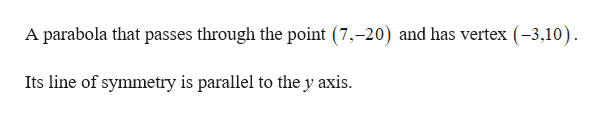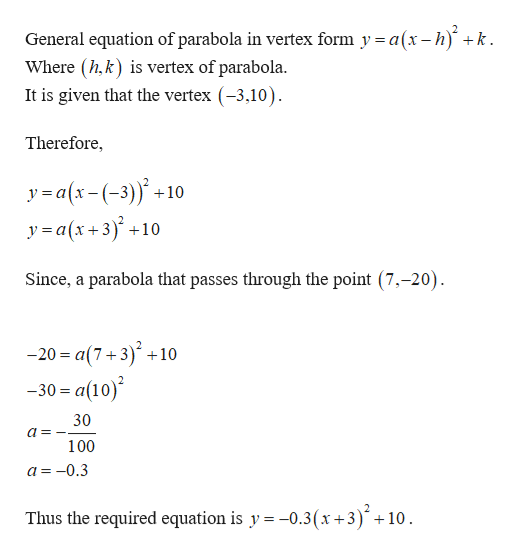# A parabola that passes through the point (7, -20) has vertex (-3, 10).  Its line of symmetry is parallel to the y-axis.Find equation of the parabola:  y =  When x = 17, what is the value of y: What is the average rate of change between x = -3 and x = 17:

Question
40 views

A parabola that passes through the point (7, -20) has vertex (-3, 10).  Its line of symmetry is parallel to the y-axis.

Find equation of the parabola:  y =

When x = 17, what is the value of y:

What is the average rate of change between x = -3 and x = 17:

check_circle

Step 1

Given information:help_outlineImage TranscriptioncloseA parabola that passes through the point (7,-20) and has vertex (-3,10) Its line of symmetry is parallel to the y axis fullscreen
Step 2

Find the equation of the parabola as follows.help_outlineImage TranscriptioncloseGeneral equation of parabola in vertex form y = a(x- h) k Where (h,k) is vertex of parabola. It is given that the vertex (-3,10) Therefore, ya(x-(-3)10 y=a(x+3)+ 10 Since, a parabola that passes through the point (7,-20). - 20 %3 а(7+3)* +10 -30 a(10) 30 100 a=-0.3 Thus the required equation is y -0.3(x +3)+ 10 fullscreen
Step 3

Find the value of y when x...

### Want to see the full answer?

See Solution

#### Want to see this answer and more?

Solutions are written by subject experts who are available 24/7. Questions are typically answered within 1 hour.*

See Solution
*Response times may vary by subject and question.
Tagged in

### Other